# RETURN ON INVESTMENT

The profits on a loan portfolio can be measured in terms of percentage return. There are three ways, widely accepted by the industry, to measure the returns on a fixed income portfolio. These measures are:

1. Simple Return of the Portfolio

2. Net Annualized Return (NAR) of the Portfolio

3. Internal Rate of Return (IRR) of the Portfolio

## Simple Return on the Portfolio

As the name suggests, Simple Return represents a ratio of profit (or loss) on the portfolio divided by the principal invested. The return so calculated does not take into account the time period over which the profit was earned. Instead, a simple calculation of profit (loss) is determined at any given time, as shown below: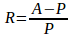where,

R = Simple rate of return

A = Value of portfolio at any given instant of time

P = Principal or investment amount

## Net Annualized Return (NAR)

Net annualized return (NAR) of a portfolio represents a compounded monthly return of the rupee cost average of monthly returns of the portfolio. The monthly income consists of interest component of the Estimate Monthly Instalment (EMI), penalty and late fee charges minus any expenses such as investment fees and losses. The monthly income so determine is divided by the principal outstanding for the month. The pay-outs are different each month, therefore, monthly returns vary month over month. NAR is calculated using the equation given below.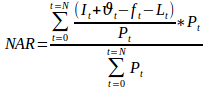where,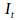= Interest earned for time period t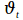= Variable income such as penalty and late fees charges earned for time period t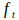= Lender fees during time period t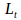= Losses during time period t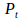= Outstanding principal during time t

Faircent calculates three variants of NAR depending on how the losses are determined. These variants are:

1. Gross NAR excluding loss – The losses are considered to be zero. There are several instances where it is difficult to determine if late payments will result in loan being written off. Losses are, therefore, considered to be zero resulting in an optimistic scenario. Interest from due payments is treated as zero but principal is not considered as a loss.

2. Optimistic NAR – Any EMI payment that is 180 days past due is a default and the principal of that month is considered to be a loss. The unpaid portion of the loan is not considered to be a loss but only the EMIs that are 180 days past due.

3. Pessimistic NAR – If any EMI of a loan is 180 days past due, the loan is considered to be unrecoverable and the unpaid principal of the loan is treated as a loss.

The NAR so calculated is highest for the Gross NAR excluding loss scenario and lowest for the pessimistic one. If all EMIs are paid then all three NARs will be same. Over time, NAR changes continuously. This is due to several factors as given below.

1. EMIs are not paid on time or maybe in default

2. Loans are foreclosed. Any foreclosed loan may attract a penalty resulting in increased income and a higher NAR.

3. NAR of a young portfolio is high. Initial EMI payments have a larger interest component, while the latter ones have a higher principal component.

## Internal Rate of Return (IRR) of the Portfolio

Internal Rate of Return (IRR) is a time sensitive calculation of an investment and is used to determine if the project is worth investing into. IRR is the interest rate at which the sum of the present value of all the cash flows, positive or negative, from the investment is zero. With reference to the loan portfolio, any return from the investment is considered to be positive cash and any disbursement of the principal is negative cash flow. IRR is a measure, unlike the first two, that takes into account the time value of money.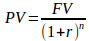where,

PV = Present value of the investment

FV = Future value of the investment

r = Internal rate of return

n = number of the years of investment

It is worth mentioning that investments are made at various times and pay-outs are also received at different times. The equation given above can be expanded as given below.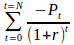+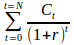= 0

where,= Principal invested at time t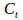= Cash received at time t

N = Number of cash flows, both positive and negative

r = Internal rate of return that satisfies the above equation

t = time in years from start at which the investment is made or cash is received